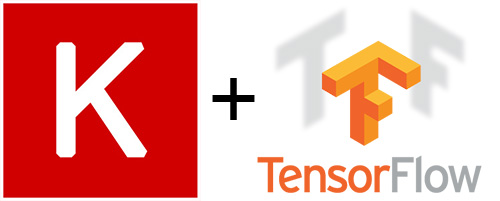# How to leverage TensorFlow's TFRecord to train Keras modelIn our previous post, we discovered how to build new TensorFlow Datasets and Estimator with Keras Model for latest TensorFlow 1.4.0. The input function takes raw image files as input. In this post, we will continue our journey to leverage Tensorflow TFRecord to reduce the training time by 21%.

I will show you

• How to turn our image files to a TFRecord file.
• Modify our input function to read the TFRecord Dataset.

Before reading on, if you haven't checkout out our previous post, it is suggested to do so. So that you are familiar with the process to turn a Keras model to a TensorFlow Estimator, and the basics of Datasets API.

## Convert Image files to TFRecord File

Once we have a list of image files and associated labels(0-Cat, 1-Dog).

```['./data/dog_vs_cat_small\\train\\cats\\cat.954.jpg'
'./data/dog_vs_cat_small\\train\\dogs\\dog.240.jpg'
'./data/dog_vs_cat_small\\train\\dogs\\dog.887.jpg'
'./data/dog_vs_cat_small\\train\\cats\\cat.770.jpg'
'./data/dog_vs_cat_small\\train\\dogs\\dog.802.jpg'
'./data/dog_vs_cat_small\\train\\dogs\\dog.537.jpg'
'./data/dog_vs_cat_small\\train\\cats\\cat.498.jpg'
'./data/dog_vs_cat_small\\train\\cats\\cat.554.jpg'
'./data/dog_vs_cat_small\\train\\cats\\cat.589.jpg'
'./data/dog_vs_cat_small\\train\\dogs\\dog.685.jpg' ...]
[0 1 1 0 1 1 0 0 0 1 ...]```

We can write the function for reading images from disk and writing them along with the class-labels to a TFRecord file.

Note that in the `convert` function, we resize all image to (150,150) so we don't have to do it in the training process like in the previous post, it also makes the generated TFRecord files' size smaller.

```def wrap_int64(value):
return tf.train.Feature(int64_list=tf.train.Int64List(value=[value]))

def wrap_bytes(value):
return tf.train.Feature(bytes_list=tf.train.BytesList(value=[value]))

def convert(image_paths, labels, out_path, size=(150,150)):
# Args:
# image_paths   List of file-paths for the images.
# labels        Class-labels for the images.
# out_path      File-path for the TFRecords output file.
print("Converting: " + out_path)
# Number of images. Used when printing the progress.
num_images = len(image_paths)
# Open a TFRecordWriter for the output-file.
with tf.python_io.TFRecordWriter(out_path) as writer:
# Iterate over all the image-paths and class-labels.
for i, (path, label) in enumerate(zip(image_paths, labels)):
# Print the percentage-progress.
print_progress(count=i, total=num_images-1)
img = Image.open(path)
img = img.resize(size)
img = np.array(img)
# Convert the image to raw bytes.
img_bytes = img.tostring()
# Create a dict with the data we want to save in the
# TFRecords file. You can add more relevant data here.
data = \
{
'image': wrap_bytes(img_bytes),
'label': wrap_int64(label)
}
# Wrap the data as TensorFlow Features.
feature = tf.train.Features(feature=data)
# Wrap again as a TensorFlow Example.
example = tf.train.Example(features=feature)
# Serialize the data.
serialized = example.SerializeToString()
# Write the serialized data to the TFRecords file.
writer.write(serialized)
```

If we call the `convert` function, it will generate the train and test TFRecord Files for us.

```convert(image_paths=train_files,
labels=train_labels,
out_path=path_tfrecords_train)
convert(image_paths=test_files,
labels=test_labels,
out_path=path_tfrecords_test)
```

## Input function to read the TFRecord Dataset

Our Estimator needs a new input function that read the TFRecord Dataset file, we call the` tf.data.TFRecordDataset` function to read the TFRecord file we created earlier.

Notice that since the image data is serialized, so we will need to turn it back to its original shape(150, 150, 3) with `tf.reshape`.

```def imgs_input_fn(filenames, perform_shuffle=False, repeat_count=1, batch_size=1):
def _parse_function(serialized):
features = \
{
'image': tf.FixedLenFeature([], tf.string),
'label': tf.FixedLenFeature([], tf.int64)
}
# Parse the serialized data so we get a dict with our data.
parsed_example = tf.parse_single_example(serialized=serialized,
features=features)
# Get the image as raw bytes.
image_shape = tf.stack([150, 150, 3])
image_raw = parsed_example['image']
label = tf.cast(parsed_example['label'], tf.float32)
# Decode the raw bytes so it becomes a tensor with type.
image = tf.decode_raw(image_raw, tf.uint8)
image = tf.cast(image, tf.float32)
image = tf.reshape(image, image_shape)
image = tf.subtract(image, 116.779) # Zero-center by mean pixel
image = tf.reverse(image, axis=) # 'RGB'->'BGR'
d = dict(zip([input_name], [image])), [label]
return d

dataset = tf.data.TFRecordDataset(filenames=filenames)
# Parse the serialized data in the TFRecords files.
# This returns TensorFlow tensors for the image and labels.
dataset = dataset.map(_parse_function)
if perform_shuffle:
# Randomizes input using a window of 256 elements (read into memory)
dataset = dataset.shuffle(buffer_size=256)
dataset = dataset.repeat(repeat_count)  # Repeats dataset this # times
dataset = dataset.batch(batch_size)  # Batch size to use
iterator = dataset.make_one_shot_iterator()
batch_features, batch_labels = iterator.get_next()
return batch_features, batch_labels
```

## Train and evaluate the model

Similar to the previous post, the `imgs_input_fn` function takes the path to the TFRecord files, and there is no parameter for the labels since they are already included in the TFRecord files.

```train_spec = tf.estimator.TrainSpec(input_fn=lambda: imgs_input_fn(path_tfrecords_train,
perform_shuffle=True,
repeat_count=5,
batch_size=20),
max_steps=500)
eval_spec = tf.estimator.EvalSpec(input_fn=lambda: imgs_input_fn(path_tfrecords_test,
perform_shuffle=False,
batch_size=1))
tf.estimator.train_and_evaluate(est_catvsdog, train_spec, eval_spec)
```

## Results

To show you the result of the training speed boost, we timed the execution of the `tf.estimator.train_and_evaluate` call.

```import time
start_time = time.time()
tf.estimator.train_and_evaluate(est_catvsdog, train_spec, eval_spec)
print("--- %s seconds ---" % (time.time() - start_time))
```

Previous post reading raw images and labels.

`--- 185.2788429260254 seconds ---`

This post with TFRecord file as Datasets.

`--- 146.32020020484924 seconds ---`

## Future work

The cat vs dog datasets we are using here is relatively small. If we are working on larger datasets that do not fit into our memory. The same`tf.data.TFRecordDataset` class we used in the post also enables us to stream the contents of more than one TFRecord file as part of an input pipeline.

```# Creates a dataset that reads all of the examples from two files.
filenames = ["/data/file1.tfrecord", "/data/file2.tfrecord"]
dataset = tf.data.TFRecordDataset(filenames)
```

Check out the full source code in my GitHub repo for this post

Tensorflow Guide - Datasets - Consuming TFRecord data

Current rating: 3.3